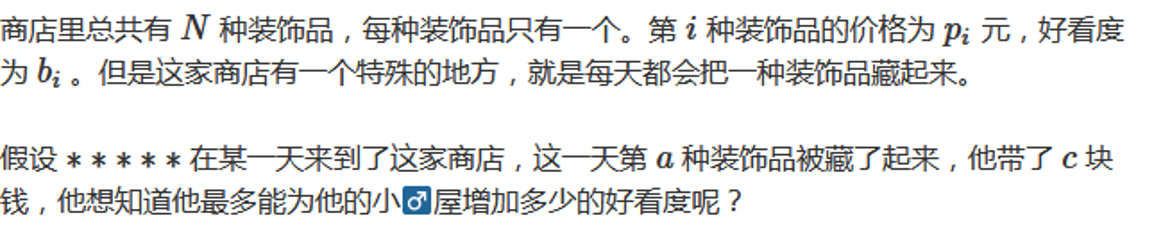# YZOJ P3033 背包

• ### 题目描述

（即每种物品按照 $$1,2,3, \cdots ,b_i$$ 编号的方案数和不编号的方案数。）

• ### 题目描述

1，$$\forall 1\le i\le m$$ 满足 $$b_i\in\mathbf{N^*}$$ 且 $$1\le b_i\le n$$

2，$$\sum_\limits{i=1}^{m}b_i\le k$$

…

# [2017-2018 Petrozavodsk WC] J. Subsequence Sum Queries

• ### 题目描述…